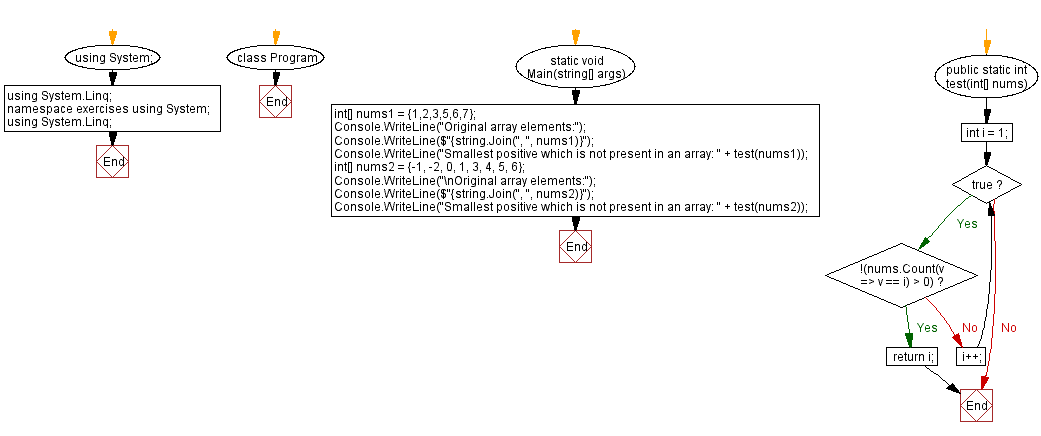﻿ C# - Smallest positive which is not present in an array# C# Sharp Exercises: Smallest positive which is not present in an array

## C# Sharp Array: Exercise-40 with Solution

Write a C# Sharp program that takes and array of integers and find the smallest positive which is not present in the said array.

Sample Data:
({ 1,2,3,5,6,7}) -> 4
({-1, -2, 0, 1, 3, 4, 5, 6}) -> 2

Sample Solution:

C# Sharp Code:

``````using System;
using System.Linq;
namespace exercises
{
class Program
{
static void Main(string[] args)
{
int[] nums1 = {1,2,3,5,6,7};
Console.WriteLine("Original array elements:");
Console.WriteLine(\$"{string.Join(", ", nums1)}");
Console.WriteLine("Smallest positive which is not present in an array: " + test(nums1));
int[] nums2 = {-1, -2, 0, 1, 3, 4, 5, 6};
Console.WriteLine("\nOriginal array elements:");
Console.WriteLine(\$"{string.Join(", ", nums2)}");
Console.WriteLine("Smallest positive which is not present in an array: " + test(nums2));
}
public static int test(int[] nums)
{
int i = 1;
while (true)
{
if (!(nums.Count(v => v == i) > 0))
return i;
i++;
}
}
}
}
```
```

Sample Output:

```Original array elements:
1, 2, 3, 5, 6, 7
Smallest positive which is not present in an array: 4

Original array elements:
-1, -2, 0, 1, 3, 4, 5, 6
Smallest positive which is not present in an array: 2
```

Flowchart:C# Sharp Code Editor:

Contribute your code and comments through Disqus.

Previous C# Sharp Exercise: Sum of all prime numbers in an array.
Next C# Sharp Exercise: Find the product of two integers in an array.

What is the difficulty level of this exercise?

Test your Programming skills with w3resource's quiz.

﻿